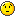# Integration of a member multi function

Hi,

I would like to integrate a member multi function func(const double* x) over 0-coordinate only (x is integration variable the rest coordinates correspond to some parameters) using ROOT::Math::IntegratorOneDim (see also attachement):

[code]#include “Math/Integrator.h”
#include “Math/WrappedFunction.h”

class MyClass{
public:
MyClass(){};
~MyClass(){};
double func(const double* x){
for(UInt_t i=0; i < 3; i++){
std::cout << " x[" << i << "] = " << x[i];
}
std::cout << std::endl;
return x+x+x;
}
typedef double (MyClass::*ForWrapper)(const double *x) ;
};

void integration(){
MyClass* myclass = new MyClass();
// double dim=3;
ROOT::Math::WrappedMemMultiFunction<MyClass,MyClass::ForWrapper> func(*myclass, &MyClass::func, 3);

``````//ROOT::Math::IntegratorOneDim integrator(ROOT::Math::IntegrationOneDim::kADAPTIVE,1.E-6,1E-4,1000, 0, Integration::GKRule::kGAUS21);

double par1 = 2.;
double par2 = -1.;
double params = {0.,par1,par2};
integrator.SetFunction(func,0,params); // integrate over 0-coordinate??
Double_t value = integrator.Integral(-1,1.);
/// integral should be equal to 2
std::cout << "Integral=" << value << " (should be equal to 2);  par1=" << par1 << "  par2=" << par2 << std::endl;
``````

};[/code]

but the result of the integration is not 1 as I would expect but 0 instead:

[code]root  .x integration.C++
Info in TUnixSystem::ACLiC: creating shared library /home/jiri/Desktop/rootProblem/integration/./integration_C.so
x = 0 x = 0 x = 0
x = -0.987993 x = 0 x = 0

x = -0.101142 x = 0 x = 0
x = 0.101142 x = 0 x = 0
Integral=0 (should be equal to 2); par1=2 par2=-1[/code]

Two parameters par1 (x) and par2 (x) are zero inside MyClass::func. Am I passing the params vector somehow wrongly to the integrator? Or is there any other reason for obtaining the wrong result? I’ve tried several possibilities but without any success…Many thanks for any useful hint how to fix it!

Cheers,
Jiri
integration.C (1.12 KB)

Hi,

Thank you for reporting this problem. It is now fixed in 5.34 patches

BTW, the integral result from the test should be 2 and not 1
Best Regards
Lorenzo

Hi,

oh, ok thanks a lot!

Is there any tag/branch I could use to test it? I don’t see special one on the list (v5-34-09 is an old one, isn’t it?):

``` git tag -l```

I’m new to git, should master branch work?

Cheers,
Jiri

(Yes, the integral should be, indeed, 2! My post was editted accordingly.)

Hi

There is no tag yet, but you can use the head of the 5.34 patch branch. After having cloned ROOT, you can do :

git checkout -b v5-34-00-patches origin/v5-34-00-patches

and you will get the head of 5.34 patches.
A tag, like 5.34.10 will be probably done in few days- one week

Cheers

Lorenzo

Hi,

the v5-34-00-patches branch works, thanks a lot!

Cheers,
Jiri